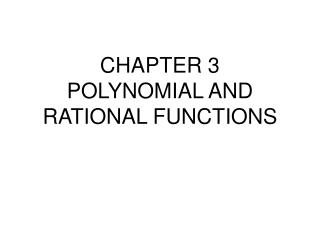DownloadDownload PresentationCHAPTER 3 POLYNOMIAL AND RATIONAL FUNCTIONS

# CHAPTER 3 POLYNOMIAL AND RATIONAL FUNCTIONS

Télécharger la présentation## CHAPTER 3 POLYNOMIAL AND RATIONAL FUNCTIONS

- - - - - - - - - - - - - - - - - - - - - - - - - - - E N D - - - - - - - - - - - - - - - - - - - - - - - - - - -
##### Presentation Transcript

1. CHAPTER 3POLYNOMIAL AND RATIONAL FUNCTIONS

2. 3.1 Quadratic Functions Objectives Recognize characteristics of parabolas Graph parabolas Determine a quadratic function’s minimum or maximum value. Solve problems involving a quadratic function’s minimum or maximum value.

3. Quadratic functionsf(x)=graph to be a parabola. The vertex of the parabolas is at (h,k) and “a” describes the “steepness” and direction of the parabola given

4. Minimum (or maximum) function value for a quadratic occurs at the vertex. If equation is not in standard form, you may have to complete the square to determine the point (h,k). If parabola opens up, f(x) has a min., if it opens down, f(x) has a max. This parabola opens up with a “steepness” of 2 and the minimum is at (1,1). (graph on next page)

5. Graph of

6. 3.2 Polynomial Functions & Their Graphs Objectives Identify polynomial functions. Recognize characteristics of graphs of polynomials. Determine end behavior. Use factoring to find zeros of polynomials. Identify zeros & their multiplicities. Use Intermediate Value Theorem. Understand relationship between degree & turning points. Graph polynomial functions.

7. The highest degree in the polynomial is the degree of the polynomial. The leading coefficient is the coefficient of the highest degreed term. Even-degree polynomials have both ends opening up or opening down. Odd-degree polynomials open up on one end and down on the other end. WHY? (plug in large values for x and see!!)

8. Zeros of polynomials When f(x) crosses the x-axis. How can you find them? Let f(x)=0 and solve. Graph f(x) and see where it crosses the x-axis. What if f(x) just touches the x-axis, doesn’t cross it, then turns back up (or down) again? This indicates f(x) did not change from positive or negative (or vice versa), the zero therefore exists from a square term (or some even power). We say this has a multiplicity of 2 (if squared) or 4 (if raised to the 4th power).

9. Intermediate Value Theorem If f(x) is positive (above the x-axis) at some point and f(x) is negative (below the x-axis) at another point, f (x) = 0 (on the x-axis) at some point between those 2 pts. True for any polynomial.

10. Turning points of a polynomial If a polynomial is of degree “n”, then it has at most n-1 turning points. Graph changes direction at a turning point.

11. Graph

12. Graph, state zeros & end behavior END behavior: 3rd degree equation and the leading coefficient is negative, so if x is a negative number such as -1000, f(x) would be the negative of a negative number, which is positive! (f(x) goes UP as you move to the left.) and if x is a large positive number such as 1000, f(x) would be the negative of a large positive number (f(x) goes DOWN as you move to the right.) ZEROS: x = 0, x = 3 of multiplicity 2 Graph on next page

13. Graph f(x)

14. Which function could possibly coincide with this graph?

15. 3.3 Dividing polynomials; Remainder and Factor Theorems Objectives Use long division to divide polynomials. Use synthetic division to divide polynomials. Evaluate a polynomials using the Remainder Theorem. Use the Factor Theorem to solve a polynomial equation.

16. How do you divide a polynomial by another polynomial? Perform long division, as you do with numbers! Remember, division is repeated subtraction, so each time you have a new term, you must SUBTRACT it from the previous term. Work from left to right, starting with the highest degree term. Just as with numbers, there may be a remainder left. The divisor may not go into the dividend evenly.

17. Remainders can be useful! The remainder theorem states: If the polynomial f(x) is divided by (x – c), then the remainder is f(c). If you can quickly divide, this provides a nice alternative to evaluating f(c).

18. Factor Theorem f(x) is a polynomial, therefore f(c) = 0 if and only if x – c is a factor of f(x). If we know a factor, we know a zero! If we know a zero, we know a factor!